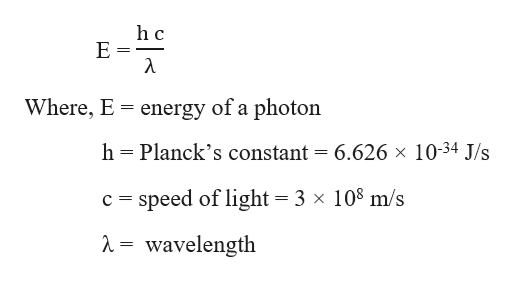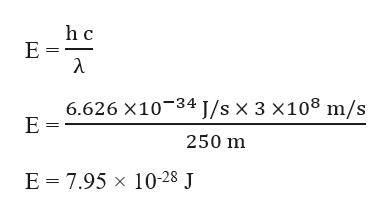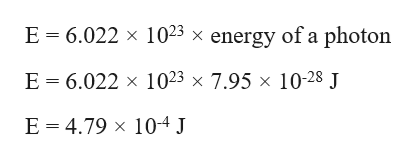# The equipment used for aviation communications emits high‑frequency radiowave energy with a wavelength of 0.250 km. What is the energy of exactly one photon of this radiowave radiation??=___________________ JWhat is the energy of one mole of this radiowave radiation??=______________ J/mol

Question
445 views

The equipment used for aviation communications emits high‑frequency radiowave energy with a wavelength of 0.250 km.

What is the energy of exactly one photon of this radiowave radiation?

?=___________________ J

?=______________ J/mol

check_circle

Step 1

The energy of a photon of a radiation is expressed as:help_outlineImage Transcriptioncloseh c E Where, E energy of a photon Planck's constant = 6.626 x 1034 J/s h c speed of light = 3 x 108 m/s A = wavelength fullscreen
Step 2

And the energy of one mole of the radiation = 6.022 × 1023 × energy of a photon

Given,

We know that, 1 km = 1000 m

⸫ wavelength in m = 0.250 × 1000 m = 250 m

The energy of one photon of this radiowave radiation can be calculated as:help_outlineImage Transcriptioncloseh c Е- λ 6.626 х10-34 ]/sx 3x108 m/s Е- 250 m E 3D 7.95 х 10-28 J fullscreen
Step 3

The energy of one mole of this radiowave&n...help_outlineImage TranscriptioncloseE 6.022 x 1023 x energy of a photon E 6.022 x 1023 x 7.95 x 10-28 J E 4.79 x 104 J fullscreen

### Want to see the full answer?

See Solution

#### Want to see this answer and more?

Solutions are written by subject experts who are available 24/7. Questions are typically answered within 1 hour.*

See Solution
*Response times may vary by subject and question.
Tagged in

### General Chemistry